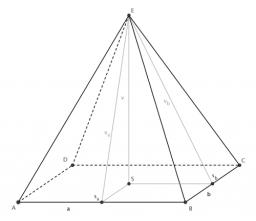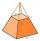# Pyramid four sides

In a regular tetrahedral pyramid is a body height of 38 cm and a wall height of 42 cm. Calculate the surface area of the pyramid; the result round to square centimeters.

S =  4285 cm2

### Step-by-step explanation:Did you find an error or inaccuracy? Feel free to write us. Thank you!Tips to related online calculators
Pythagorean theorem is the base for the right triangle calculator.

#### You need to know the following knowledge to solve this word math problem:

We encourage you to watch this tutorial video on this math problem:

## Related math problems and questions:In a regular quadrilateral pyramid, the height is 6.5 cm and the angle between the base and the side wall is 42°. Calculate the surface area and volume of the body. Round calculations to 1 decimal place.
• Tetrahedral pyramidCalculate the volume and surface area of a regular tetrahedral pyramid, its height is \$b cm and the length of the edges of the base is 6 cm.
• Tetrahedral pyramidWhat is the surface of a regular tetrahedral (four-sided) pyramid if the base edge a=16 and height v=16?
• Tetrahedral pyramidCalculate the surface S and the volume V of a regular tetrahedral pyramid with the base side a = 5 m and a body height of 14 m.
• Pyramid 4sidesCalculate the volume and the surface of a regular quadrangular pyramid when the edge of the base is 4 cm long and the height of the pyramid is 7 cm.Find the height and surface of a regular quadrilateral pyramid with a base edge a = 8cm and a wall height w = 10cm. Sketch a picture.
• Regular triangular pyramidCalculate the volume and surface area of the regular triangular pyramid and the height of the pyramid is 12 centimeters, the bottom edge has 4 centimeters and the height of the side wall is 12 centimeters
• Find theFind the surface area of a regular quadrilateral pyramid which has a volume of 24 dm3 and a height of 45 cm.Given is a regular quadrangular pyramid with a square base. The body height is 30 cm and volume V = 1000 cm³. Calculate its side a and its surface area.
• PillarCalculate volume of pillar shape of a regular tetrahedral truncated pyramid, if his square have sides a = 19, b = 27 and height is h = 48.Find the volume and surface of a regular quadrilateral pyramid if the bottom edge is 45 cm long and the pyramid height is 7 cm.Calculate the regular tetrahedral pyramid's volume and surface if the content area of the base is 20 cm2, and the deviation angle of the side edges from the plane of the base is 60 degrees.Determine the surface of a regular tetrahedral pyramid when its volume is V = 120 and the angle of the sidewall with the base plane is α = 42° 30'.We have a regular quadrilateral pyramid with a base edge a = 10 cm and a height v = 7 cm. Calculate 1/base content 2/casing content 3/pyramid surface 4/volume of the pyramidIt is given a regular tetrahedral pyramid with a base edge of 6 cm and the height of the pyramid 10 cm. Calculate the length of its side edges.Calculate the surface and volume of a regular quadrangular pyramid if side a = 6 cm and wall height v = 0.8dm.Calculate the surface area of a regular hexagonal pyramid with a base inscribed in a circle with a radius of 8 cm and a height of 20 cm.